Magnetic Field Around Circular Wire

Magnetic Field around a Circular Wire

Circular wire produces magnetic field inside the circle and outside the circle. Magnetic field around a circular wire is calculated by the formula;

B=2πk.i/r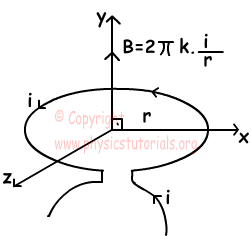Direction of the magnetic field at the center of the circle is found with right hand rule.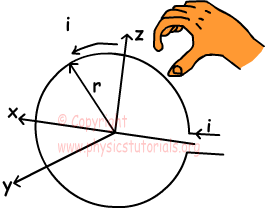Your thumb shows the direction of magnetic field and four fingers show direction of current. Moreover, we can show the direction of current inside the circle with following pictures;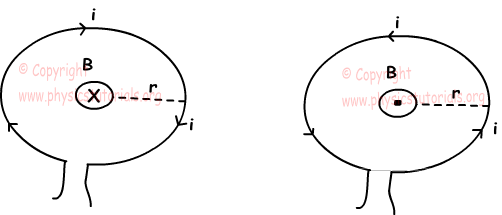Example: Find the magnitude and direction of magnetic field at the center of the semicircle given below.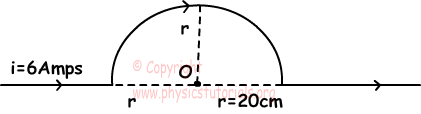When we apply right hand rule we see that direction of magnetic field is inward to the page as shown in the picture below, since we have semicircle, we put 1/2 in front of our formula;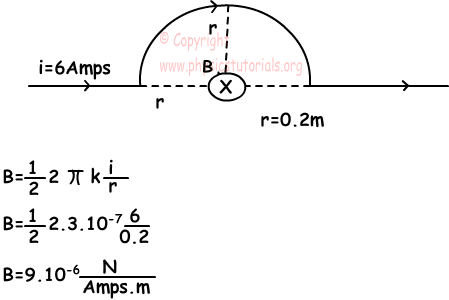Example: Directions of i1 and i2 currents are opposite. If the magnetic field at the center of the circles is zero find the ratio of i1 to i2 i1/i2?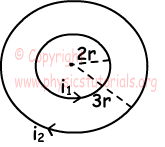Smaller circle has magnetic field ;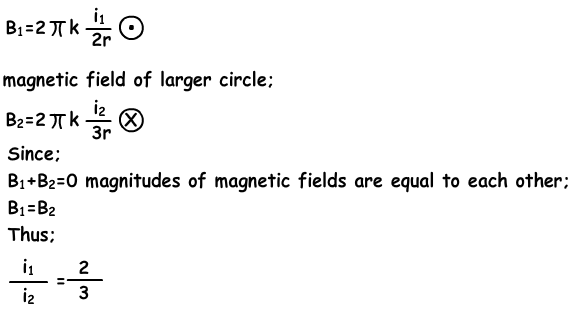Example: Find the magnetic field produced by currents i1 and i2 at point O.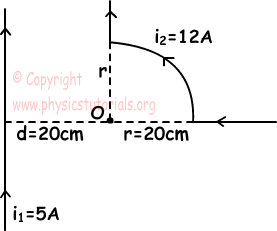If we apply right hand rule, directions of currents are;

i1:inward

i2:outward

Thus, total magnetic field at point O becomes the difference of these magnetic fields.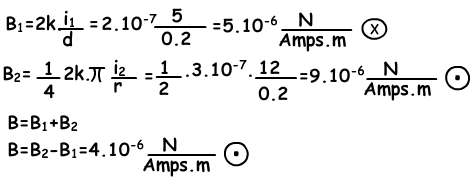Magnetism Exams and Solutions

Author:

Tags: